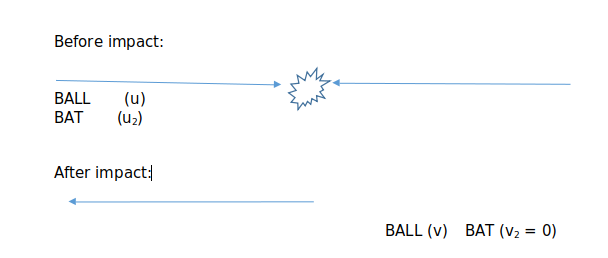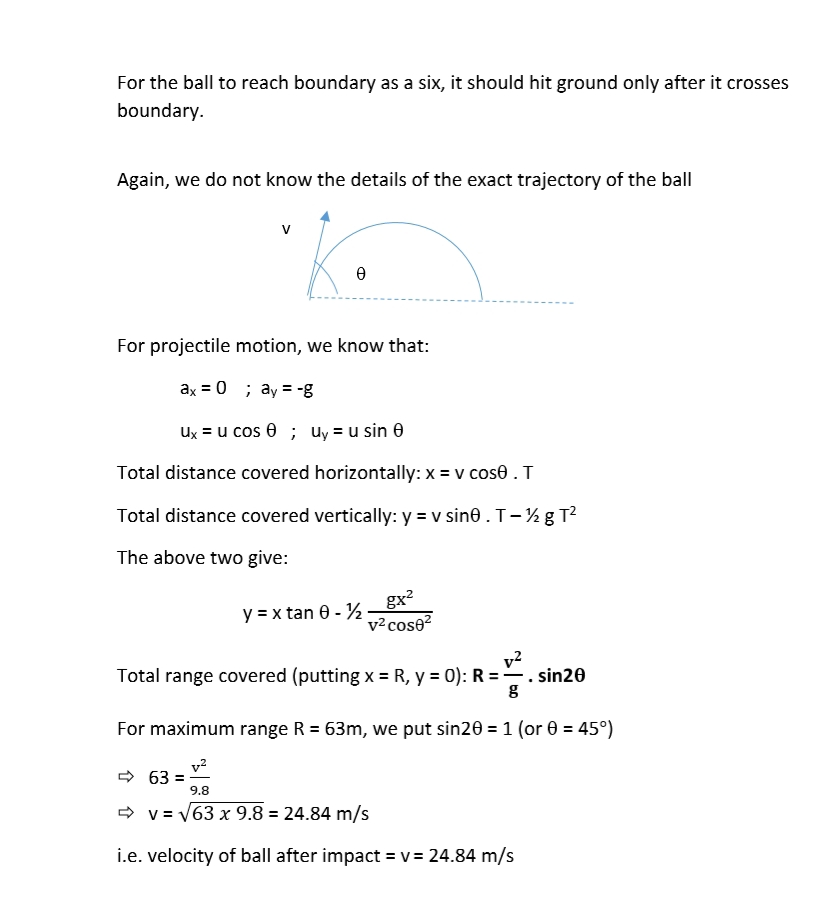# Super 30 Movie - Maths QuizHere is some fun time! Can you solve all the questions asked in Super 30 movie released recently?

Let us give them a try here.

Q1: There are 30 students in a class. If every students shakes hands with every other student, such that no students shake each other’s hands more than once, then what shall be the total number of handshakes?

Q2: At such high altitudes and at -35°C, why does water not become ice in the clouds?

Q3: There are 30 students in a class. If I give one egg to the first student, two eggs to the second student, four eggs to the third student, and so on, then how many eggs will the last student get?

Q4: If a train is moving at a speed of 120 km/hr, and I start removing one coach at a time till all of them are removed, then what shall be the final speed of the engine?

Q5: In a cricket match, if Sohaib Akhtar throws the ball at 143 km/hr, and the boundary is 63 meters away, then with what force should Sachin Tendulkar hit the ball to score a six?

Look at the solutions below only after you have tried solving all of the above.

SOLUTIONS

1. There are two approaches to solve this:

Approach 1:

Permutation/Combination approach:

There are 30 students and we have to pick 2 at a time such that there are no repetitions.

Formula to apply:

nCr = n! / r! (n - r)!

No. of handshakes = 30C2 =  30!/2!28! = (30 x 29)/2 = 435

Approach 2:

First student shakes hands with 29 remaining students

Second student now shakes hands with remaining 28 students

Third student now shakes hands with remaining 27 students

.... (and so on)

Second last student shakes hand with remaining last student

Total handshakes

= 29 + 28 + 27 + ... + 1

= 1 + 2 + 3 + ... + 29

Now: Sum of first n natural numbers = n (n + 1)/2

Therefore: No. of handshakes = (29 x 30)/2 = 435

2.

This is a phenomenon called as “super cooling”. This allows a liquid to stay in its liquid state even below its freezing point (and not solidify). This needs that there should be no nucleation centres (or seed crystals). In order for liquid water to condense to a solid, it has to be cold enough andhave somewhere to freeze onto. All it takes is a bit of dust or even vibrations to kick start crystallization. But in certain situations in the atmosphere, such nucleation centers are sometimes hard to come by. As a result, the water can stay liquid below freezing temperatures.

However, it is important to note that clouds are not always drops of liquid water. Clouds can also be composed of ice crystals.

3.

This forms a Geometric Progression

G.P. Series = 1, 2, 4, 8, 16, 32, ...   (total 30 terms)

Of the form: G.P. Series = a, ar, ar2, ar3, ar4, .... , arn-1 for n terms

Here: a = 1, r = 2, n = 30

No. of eggs given to the last student

= the nth term of the above G.P. series

= 30th term

= 1 x 230-1

= 229

= 210 x 210 x 29

= 1024 x 1024 x 512

= 536,870,912

4.

Here the train is moving at a constant speed of 120 km/hr without any acceleration.

So, the change in total mass has no effect on the speed.

The final speed = 120 km/hr

5.

Here we shall assume the standard weight (mass) of a cricket ball = 163 g

(since it is not given in the question)

Disclaimer: There is only limited information given in the question. There are multiple principles of Physics that could be applied. We will try to eliminate them by just oversimplifying the given problem. There may exist alernate approaches to this question with a very different set of assumptions.

First thing is to note that the speed is given in km/hr and distance in m.

So, first step is to convert speed into m/s

Speed of ball = u = 143 km/hr = 143 x  m/s = 39.72 m/s

Mass of ball = m = 163 g

Distance to cover = s = 63 m

initial velocity of ball before impact with bat = u = 39.72 m/s

velocity of ball after impact with bat = vApproach 1:

Force applied on ball = F =  m (v - u)/t

where:

m = mass of ball = 163 g = 0.163 kg

u = initial velocity of ball = 39.72 m/s

v = final velocity of ball = 24.84 m/s

=> F = 0.163(24.84 + 39.72)/t N               [note that v and u are in reverse directions]

=> F = 10.52/t N

However, here we do not know the time of total impact with the bat.

Approach 2:

Work done = Force x displacement = change in kinetic energy

[by law of conservation of energy]

where:

Displacement = s = 63 m

Mass of ball = m = 163 g = 0.163 kg

Initial velocity of ball before impact = u = 39.72 m/s

Final velocity of ball after impact = v = 24.84 m/s

Change in kinetic energy

= ½ m (v2 – u2

= ½ x 0.163 ((24.84)2 – (39.72)2)

= - ½ x 0.163 x 960.65

= -78.29 J

=> F x 63 = 78.29

=> F = 78.29/63 = 1.24 N